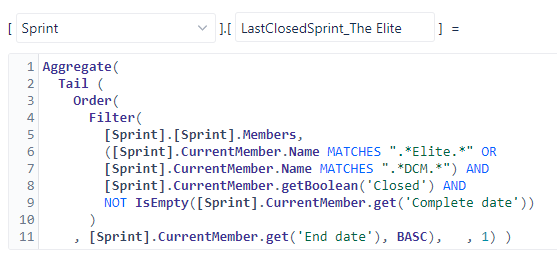# How to Combine Multiple Sprint Dimension Calculated Members

I have 10 teams each with their own JIRA project and Sprint naming convention. I pull all of these JIRA projects into 1 eazyBI account. For each team, I have created a calculated member in the Sprint Dimension that filters for that team’s last closed sprint (image attached).

I now want to gather these 10 last closed sprints so I can get totals and total averages.

I’m stuck as I don’t know a way to combine these 10 calculated members in the Sprint Dimension into a new calculated member. How do I do this?Hi,

Formula like this could be used to aggregate last closed sprints for each team if the Sprint naming conventions are followed:

``````Aggregate(
{
--team DCM
Order(
Filter([Sprint].[Sprint].Members,
[Sprint].CurrentHierarchyMember.Name MATCHES ".*DCM.*" AND
[Sprint].CurrentHierarchyMember.getBoolean('Closed') AND
NOT IsEmpty([Sprint].CurrentHierarchyMember.get('Complete date'))),
[Sprint].CurrentHierarchyMember.get('End date'),
BDESC
).Item(0),
--team Elite
Order(
Filter([Sprint].[Sprint].Members,
[Sprint].CurrentHierarchyMember.Name MATCHES ".*Elite.*" AND
[Sprint].CurrentHierarchyMember.getBoolean('Closed') AND
NOT IsEmpty([Sprint].CurrentHierarchyMember.get('Complete date'))),
[Sprint].CurrentHierarchyMember.get('End date'),
BDESC
).Item(0)
}
)
``````

Kindly,
Janis, eazyBI support